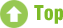## Nested if/then Statements for Everyday Use

October 19th, 2017

Logic that goes beyond the spreadsheet....

Today’s post is by Mike Figueroa, Assistant Director of Customer Services at SafeSourcing

Most problems don’t have solutions as simple as yes or no, such as “if A, then ‘yes’. If B, then ‘no’”. Solutions are more often evaluated through multiple sets of dependencies, such as “if A+B is greater than C, then find the average of X-Z”. Of course these statements aren’t intuitive, so if we don’t understand how to use them formally in logic equations or computer code, we are much less likely to get them right in real life situations. So here’s a crash course in Excel “IF” statements:

The basic description of the “IF” function is “If something is True, then do something, otherwise do something else”. To see it in action, start with typing “=IF” in an Excel cell of your choice. You’ll see Excel suggest the full formula as “=IF(logical_test, [value_if_true], [value_if_false])”. Each of those statements within the parentheses is a variable that does something specific, and they have to be separated by a comma so that Excel knows when you are entering a new variable. Here is how each variable behaves within the function:

• “logical_test”: This means there must be an active function. Let’s go with an example of “A1>B1”.
• “value_if_true”: Whatever value you include in this variable, will populate the cell you’re programing the formula for, if the “logical_test” variable is true. Such as if A1 = 2, and B1 = 1.
• “value_if_false”: Whatever value is in the false variable, will be populated if “logical_test” is untrue. Such as if A1 = 1 and B1 = 2.

So if we insert some example variables into the formula like so: “IF(2>1, “Yes”, “No”)

This function would insert a “Yes” into the cell being programmed, and is one of the more simple ways to use the function. However, you can swap any of the aforementioned variables for other formulas as well. This is called “nesting”.

For example, you could write a formula like this: “=IF(K5<K6,”YES”,IF((COUNT(H7:H10>2)),”HIGH”,”LOW”))

Let’s describe what this function is doing in sentence form, with a context of evaluating vendor proposals: If Vendor 1’s cost is lower than Vendor 2’s cost, enter “YES” into the cell, if Vendor 1 is not lower than Vendor 2, then if the number of DC’s the new vendor carries is greater than 2, enter “HIGH” into the cell, otherwise enter “LOW” into the cell. This tells me either to select Vendor 1, or to evaluate several other dependencies to make a final decision.

This level of specificity is not normal in everyday speech, but is absolutely critical in procurement. If you have a vendor proposing an agreement, a full evaluation of the value proposition can’t be based on just one variable. Practicing this and other logic functions in Excel and other tools is an excellent way to hone your ability to evaluate complex procurement problems.

For more information on how SafeSourcing can assist your team with this process or on our “Risk Free” trial program, please contact a SafeSourcing Customer Service Representative. We have an entire customer services team waiting to assist you today.

If you thought this page is useful to your friend, use this form to send.
Friend Email
Enter your message